## Total internal reflection, and Lenses

7-28-99

### Total internal reflection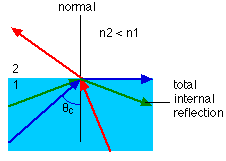When light crosses an interface into a medium with a higher index of refraction, the light bends towards the normal. Conversely, light traveling across an interface from higher n to lower n will bend away from the normal. This has an interesting implication: at some angle, known as the critical angle, light travelling from a medium with higher n to a medium with lower n will be refracted at 90°; in other words, refracted along the interface. If the light hits the interface at any angle larger than this critical angle, it will not pass through to the second medium at all. Instead, all of it will be reflected back into the first medium, a process known as total internal reflection.

The critical angle can be found from Snell's law, putting in an angle of 90° for the angle of the refracted ray. This gives: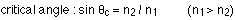For any angle of incidence larger than the critical angle, Snell's law will not be able to be solved for the angle of refraction, because it will show that the refracted angle has a sine larger than 1, which is not possible. In that case all the light is totally reflected off the interface, obeying the law of reflection.

Optical fibers are based entirely on this principle of total internal reflection. An optical fiber is a flexible strand of glass. A fiber optic cable is usually made up of many of these strands, each carrying a signal made up of pulses of laser light. The light travels along the optical fiber, reflecting off the walls of the fiber. With a straight or smoothly bending fiber, the light will hit the wall at an angle higher than the critical angle and will all be reflected back into the fiber. Even though the light undergoes a large number of reflections when traveling along a fiber, no light is lost.

### Depth perception

Because light is refracted at interfaces, objects you see across an interface appear to be shifted relative to where they really are. If you look straight down at an object at the bottom of a glass of water, for example, it looks closer to you than it really is. Looking perpendicular to an interface, the apparent depth is related to the actual depth by: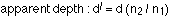### An example

A beam of light travels from water into a piece of diamond in the shape of a triangle, as shown in the diagram. Step-by-step, follow the beam until it emerges from the piece of diamond.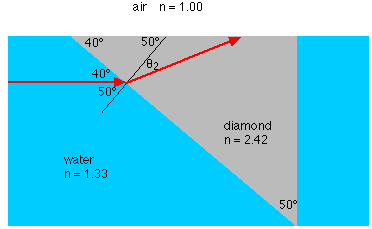(a) How fast is the light traveling inside the piece of diamond?

The speed can be calculated from the index of refraction: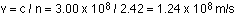(b) What is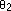, the angle between the normal and the beam of light inside the diamond at the water-diamond interface?

A diagram helps for this. In fact, let's look at the complete diagram of the whole path, and use this for the rest of the questions.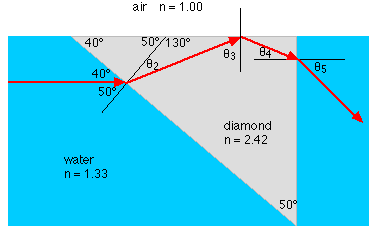The angle we need can be found from Snell's law: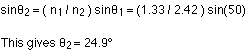(c) The beam travels up to the air-diamond interface. What is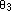, the angle between the normal and the beam of light inside the diamond at the air-diamond interface?

This is found using a bit of geometry. All you need to know is that the sum of the three angles inside a triangle is 180°. Ifis 24.9°, this means that the third angle in that triangle must be 25.1°. So: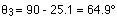(d) What is the critical angle for the diamond-air interface?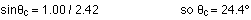(e) What happens to the light at the diamond-air interface?

Because the angle of incidence (64.9°) is larger than the critical angle, the light is totally reflected internally.

(f) The light is reflected off the interface, obeying the law of reflection. It then strikes the diamond-water interface. What happens to it here?

Again, the place to start is by determining the angle of incidence,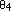. A little geometry shows that: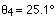The critical angle at this interface is :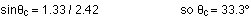Because the angle of incidence is less than the critical angle, the beam will escape from the piece of diamond here. The angle of refraction can be found from Snell's law:### Lenses

There are many similarities between lenses and mirrors. The mirror equation, relating focal length and the image and object distances for mirrors, is the same as the lens equation used for lenses.There are also some differences, however; the most important being that with a mirror, light is reflected, while with a lens an image is formed by light that is refracted by, and transmitted through, the lens. Also, lenses have two focal points, one on each side of the lens.

The surfaces of lenses, like spherical mirrors, can be treated as pieces cut from spheres. A lens is double sided, however, and the two sides may or may not have the same curvature. A general rule of thumb is that when the lens is thickest in the center, it is a converging lens, and can form real or virtual images. When the lens is thickest at the outside, it is a diverging lens, and it can only form virtual images.

Consider first a converging lens, the most common type being a double convex lens. As with mirrors, a ray diagram should be drawn to get an idea of where the image is and what the image characteristics are. Drawing a ray diagram for a lens is very similar to drawing one for a mirror. The parallel ray is drawn the same way, parallel to the optic axis, and through (or extended back through) the focal point. The chief difference in the ray diagram is with the chief ray. That is drawn from the tip of the object straight through the center of the lens. Wherever the two rays meet is where the image is. The third ray, which can be used as a check, is drawn from the tip of the object through the focal point that is on the same side of the lens as the object. That ray travels through the lens, and is refracted so it travels parallel to the optic axis on the other side of the lens.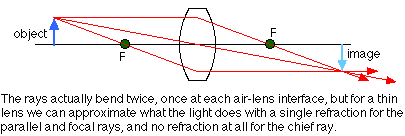The two sides of the lens are referred to as the object side (the side where the object is) and the image side. For lenses, a positive image distance means that the image is real and is on the image side. A negative image distance means the image is on the same side of the lens as the object; this must be a virtual image.

Using that sign convention gives a lens equation identical to the spherical mirror equation: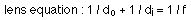The other signs work the same way as for mirrors. The focal length, f, is positive for a converging lens, and negative for a diverging lens.

The magnification factor is also given by the same equation: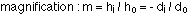Useful devices like microscopes and telescopes rely on at least two lenses (or mirrors). A microscope, for example, is a compound lens system with two converging lenses. One important thing to note is that with two lenses (and you can extend the argument for more than two), the magnification factor m for the two lens system is the product of the two individual magnification factors.

It works this way. The first lens takes an object and creates an image at a particular point, with a certain magnification factor (say, 3 times as large). The second lens uses the image created by the first lens as the object, and creates a final image, introducing a second magnification factor (say, a factor of seven). The magnification factor of the final image compared to the original object is the product of the two magnification factors (3 x 7 = 21, in this case).

### Ray diagram for a diverging lens

Consider now the ray diagram for a diverging lens. Diverging lenses come in a few different shapes, but all diverging lens are fatter on the edge than they are in the center. A good example of a diverging lens is a bi-concave lens, as shown in the diagram. The object in this case is beyond the focal point, and, as usual, the place where the refracted rays appear to diverge from is the location of the image. A diverging lens always gives a virtual image, because the refracted rays have to be extended back to meet.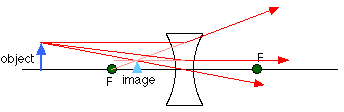Note that a diverging lens will refract parallel rays so that they diverge from each other, while a converging lens refracts parallel rays toward each other.

### An example

We can use the ray diagram above to do an example. If the focal length of the diverging lens is -12.0 cm (f is always negative for a diverging lens), and the object is 22.0 cm from the lens and 5.0 cm tall, where is the image and how tall is it?

Working out the image distance using the lens equation gives: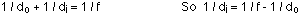This can be rearranged to:The negative sign signifies that the image is virtual, and on the same side of the lens as the object. This is consistent with the ray diagram.

The magnification of the lens for this object distance is: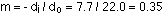So the image has a height of 5 x 0.35 = 1.75 cm.

### Sign convention

The sign convention for lenses is similar to that for mirrors. Again, take the side of the lens where the object is to be the positive side. Because a lens transmits light rather than reflecting it like a mirror does, the other side of the lens is the positive side for images. In other words, if the image is on the far side of the lens as the object, the image distance is positive and the image is real. If the image and object are on the same side of the lens, the image distance is negative and the image is virtual.

For converging mirrors, the focal length is positive. Similarly, a converging lens always has a positive f, and a diverging lens has a negative f.

The signs associated with magnification also work the same way for lenses and mirrors. A positive magnification corresponds to an upright image, while a negative magnification corresponds to an inverted image. As usual, upright and inverted are taken relative to the orientation of the object.

Note that in certain cases involving more than one lens the object distance can be negative. This occurs when the image from the first lens lies on the far side of the second lens; that image is the object for the second lens, and is called a virtual object.